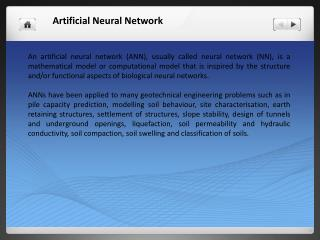DownloadDownload PresentationArtificial Neural Network

# Artificial Neural Network

Download Presentation## Artificial Neural Network

- - - - - - - - - - - - - - - - - - - - - - - - - - - E N D - - - - - - - - - - - - - - - - - - - - - - - - - - -
##### Presentation Transcript

1. An artificial neural network (ANN), usually called neural network (NN), is a mathematical model or computational model that is inspired by the structure and/or functional aspects of biological neural networks. ANNs have been applied to many geotechnical engineering problems such as in pile capacity prediction, modelling soil behaviour, site characterisation, earth retaining structures, settlement of structures, slope stability, design of tunnels and underground openings, liquefaction, soil permeability and hydraulic conductivity, soil compaction, soil swelling and classification of soils. Artificial Neural Network

2. Artificial Neural Network Three layer neural network

3. Graphical presentation of neuron in ANN

4. There are several types of architecture of NNs. However, the two most widely used NNs Feed forward networks and Recurrent networks. In a feed forward network, information flows in one direction along connecting pathways, from the input layer via the hidden layers to the final output layer. There is no feedback (loops) i.e., the output of any layer does not affect that same or preceding layer. Feed-forward neural networks, where the data ow from input to output units is strictly feedforward. The data processing can extend over multiple (layers of) units, but no feedback connections are present.

5. Variable selection • Formation of training, testing and validation sets • Neural network architecture • Evaluation criteria • Neural network training Black propagation network

6. Rock Properties Compressive strength as input Cohesion & friction angle as output Trained Data by ANN Calculate the Cohesion & Friction by input Compressive Strength Input the data in Software Analysis of Slope Stability Flow Chart to determine the properties and analysis the Slope stability by ANN

7. Fuzzy logic is a form of many-valued logic and it deals with reasoning that is approximate rather than fixed and exact. The nature of uncertainty in a slope design is a very important that should considered. Fuzzy set theory was developed specially to deal with uncertainties that are nonrandom in nature • There are several FISs that have been employed in various applications. The most commonly used include: • Mamdani Fuzzy Model; • Takagi-Sugeno-Kang fuzzy (TSK) model; • Tsukamoto fuzzy model; • Singleton fuzzy model. Fuzzy Inference System